# Hausdorff dimension

(diff) ← Older revision | Latest revision (diff) | Newer revision → (diff)
Jump to: navigation, search

A numerical invariant of metric spaces, introduced by F. Hausdorff in . Letbe a metric space. For realand, let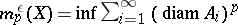, where the lower bound is taken over all countable coverings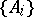offor which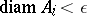. The Hausdorff dimension ofis defined as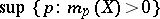, where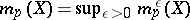. The number thus defined depends on the metric on(on this, see also Metric dimension) and is, generally speaking, not an integer (for example, the Hausdorff dimension of the Cantor set is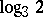). A topological invariant is, for example, the lower bound of the Hausdorff dimensions over all metrics on a given topological space; whenis compact, this invariant is the same as the Lebesgue dimension of.